Depending on what level you are at, there are some more advanced methods for finding the GCF.  This first one is probably at the pre-algebra level and the others may not be.

The Prime Factorization Method:

Let's just do one that we did the other way and I'll walk you through it:

 FindList the prime factorization of each using exponents: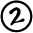Take the SMALLER of each of the exponents...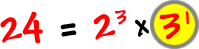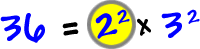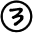Multiply these together to get the answer: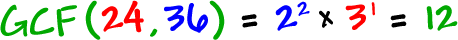Is this what we got the other way?

Yep!